# Conditional Probability

and Independence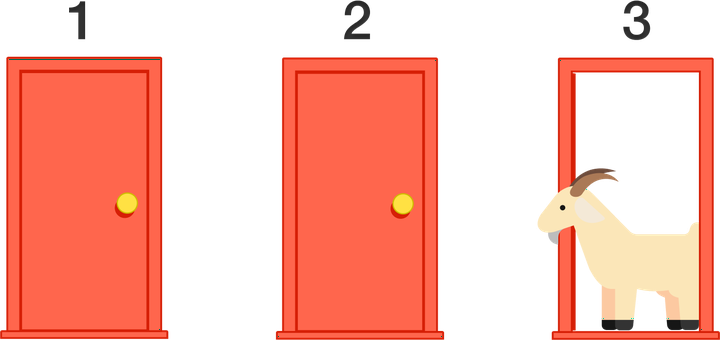For any two events A and B, with $$\mathbb{P}(B) > 0$$, we define the conditional probability of A given B ($$\mathbb{P}(A|B)$$) as

$\mathbb{P}(A|B) = \frac{\mathbb{P}(A \cap B)}{\mathbb{P}(B)}$

Example: We throw a dice once, and it lands on an even number. What is the probability of this number is 2?

We can define:

• $$A$$ = dice shows number 2
• $$B$$ = dice result is even

We have then that A = {2}, B = {2, 4, 6} and $$A \cap B = \{2\}$$, then

$\mathbb{P}(A|B) = \frac{\mathbb{P}(A \cap B)}{\mathbb{P}(B)} = \frac{1}{3}$

## Independence

Independence between two or more variables can help us to ease some of our analysis. We say that two events A and B are independent if and only if $$\mathbb{P}(A \cap B) = \mathbb{P}(A) \mathbb{P}(B)$$, i.e., two events are independent if the incidence of one event does not affect the probability of the other event. If the incidence of one event does affect the probability of the other event, then the events are dependent.

The concept of conditional probability is closely related to independent events, and we can define independence between events using conditional probability.

Two events $$A$$ and $$B$$ are independent if:

$\mathbb{P}(A\mid B) = \mathbb{P}(A \mid B^c) \text{ and } \mathbb{P}(B\mid A) = \mathbb{P}(B\mid A^c)$

Two events $$A$$ and $$B$$ are dependent if :

$\mathbb{P}(A\mid B) \neq \mathbb{P}(A \mid B^c) \text{ or } \mathbb{P}(B\mid A) \neq \mathbb{P}(B\mid A^c)$

Example: Two fair 6-sided dice are rolled, one red and one blue. $$A$$ = the red die’s result is 3. $$B$$ = the blue die’s result is 4. $$C$$ = the event that the sum of the rolls is 7. Are $$A$$, $$B$$ and $$C$$ mutually independent?

$\mathbb{P}(A|B) = \frac{1}{6} = \mathbb{P}(A \mid B^c) \implies \text{A and B are independent}$

$\mathbb{P}(A|C) = \frac{1}{6} = \mathbb{P}(A \mid C^c) \implies \text{A and C are independent}$

$\mathbb{P}(B|C) = \frac{1}{6} = \mathbb{P}(B \mid C^c) \implies \text{B and C are independent}$

Theses events are pairwise independent. However, in order for all three events to be mutually independent, each event must be independent with each intersection of the other events.

$\mathbb{P}\left(A\mid (B\cap C)\right) = 1 \text{ and } P\left(A\mid (B\cap C)^c\right)=\dfrac{1}{7}$

These are not equal, $$A$$, $$B$$, and $$C$$ are mutually dependent.

# Bayes theorem

One of the most essential conditional probability entities is the one from the Bayes theorem. The simplified formula is given by:

$\mathbb{P}(A \mid B) = \frac{\mathbb{P}(B \mid A)\mathbb{P}(A)}{\mathbb{P}(B)}$

A bit of the history of this formula and of Bayes itself. In the 1700s, the theory of Probability was consolidating itself, and we could only calculate probabilities of effects knowing the cause,

$\mathbb{P}(\text{effect} \mid \text{cause})$

The British reverend Thomas Bayes wanted to know how to infer causes from effects, i.e., he was interested in what we call the inverse probability. His question could be summarized as ‘How could we learn the probability of a future event occurring if we only knew how many times it had happened or not in the past?’, so he was interested at

$\mathbb{P}(\text{cause} \mid \text{effect})$

To illustrate this, he created an experiment. In his experiment, Bayes asked his assistant to drop the ball on a table when his back was turned to the table.

The table is flat, so the ball has just as much chance of landing at any one place on the table as anywhere else. Now Bayes has to figure out where the ball is, without looking.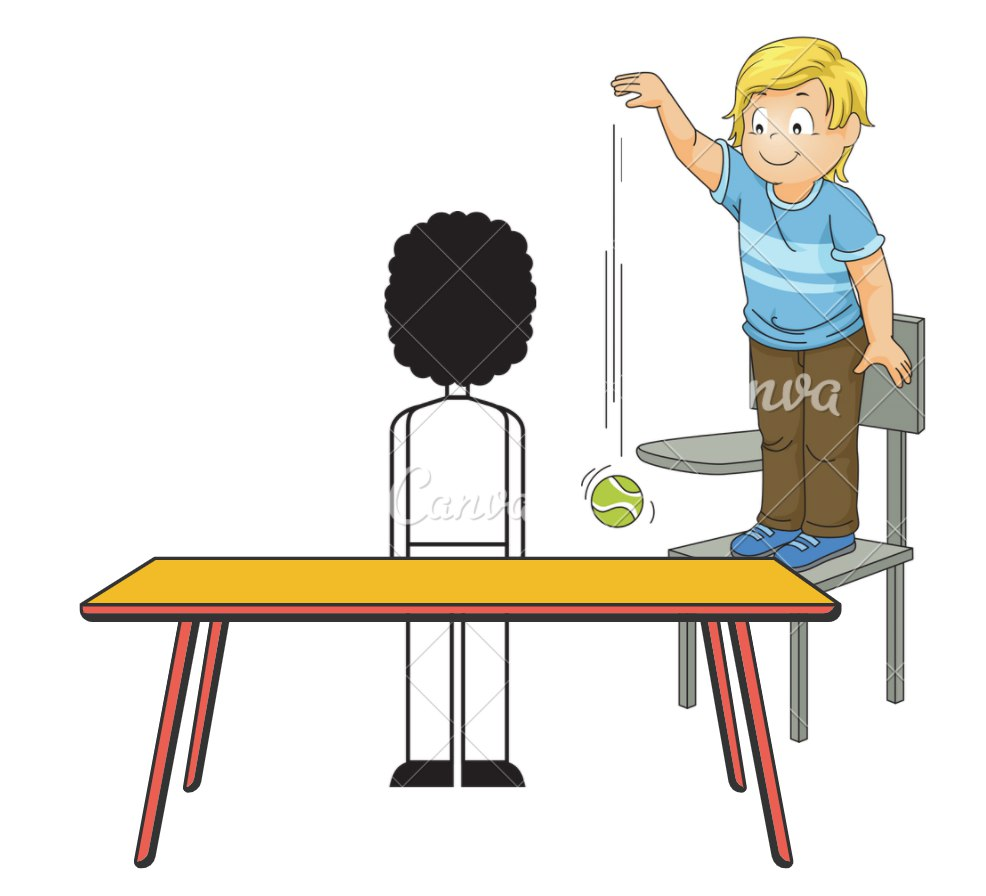He then asks his assistant to throw another ball on the table and to tell him if the new ball is to the left or right to the first ball. Where he could conclude:

• If the new ball landed to the left of the first ball, then the first ball is more likely to be on the right side of the table than the left side.

He asks his assistant to throw another ball. If it again lands to the left of the first ball, then the first ball is even more likely than before to be on the right side of the table. And so on.

The more balls we throw, the more we can narrow down where the first ball probably is. Each new piece of information constrains the area where the first ball probably is.

This is a learning process!

Before having an observation, any position is as possible as any other. Bayes system shows us that an initial belief with data gives us an improved view.

• The initial belief is what we call prior
• The improved opinion is the posterior

In each new round of belief updating, the most recent posterior becomes the prior for the new calculation.

Bayes never published his discovery, his friend Richard Price found it among his notes after Bayes’ death in 1761, re-edited it, and published it. Bayes also did not create modern concepts such as Bayesian statistics or Bayesian inference. These were introduced in the 1950s.

There were two criticisms to Bayes’ system:

1. Mathematicians disapproved the use of guessing in rigorous mathematics.
2. Bayes said that if he didn’t know what guess to make, he’d just assign all possibilities equal.

Pierre-Simon Laplace, a brilliant young mathematician, rediscovered Bayes’ mechanism and published it in 1774. Laplace stated that the probability of a cause (given an event) is proportional to the probability of the event (given its cause). The formula and all concepts regarding Bayes theorem and eventually Bayesian Statistic are due to the work of Laplace.

We can generalise the results of the theorem by assuming that $$\{C_1, C_2, …, C_n\}$$ are partitions of the sample space $$\Omega$$, i.e.,

$C_i \cap C_j = \varnothing \text{ if } i=j$ $C_1 \cup C_2 \cup … \cup C_n = \Omega$

If we consider some event $$A$$, where $$A \in \Omega$$ with know probabilities $$\mathbb{P}(C_i)$$ and $$\mathbb{P}(A \mid C_i)$$ we result in the full version of the theorem. The picture below demonstrates this.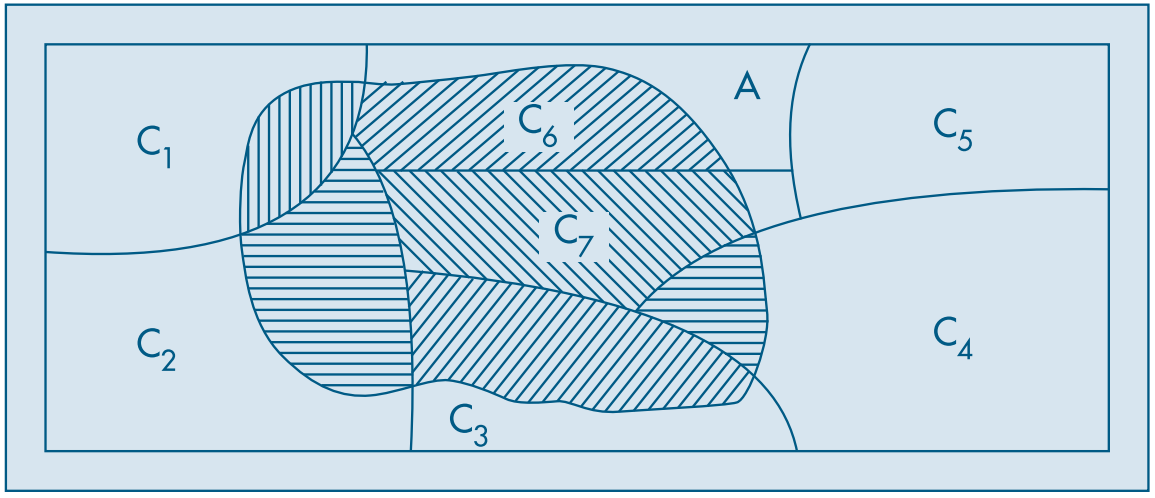The full version of the theorem is the probability of occurrence of an event $$C_i$$ given that the event A has happened is given by:

$\mathbb{P}(C_i \mid A) = \frac{\mathbb{P}(C_i)\mathbb{P}(A \mid C_i)}{\sum_{j=1}^n\mathbb{P}(C_j)\mathbb{P}(A \mid C_j)}, \forall i=1,2,…, n$

We can think of $$C_1, C_2, …, C_n$$ as a set of hypotheses, where only one of them is true. Given that A occurred, the initial probability of $$C_i$$ is is updated by the extra knowledge.

A very famous problem that we can solve using Bayes theorem is the Monty Hall. The Monty Hall problem is based on the American television game show Let’s Make a Deal and named after its host, Monty Hall.

Suppose you’re on a game show, and you’re given the choice of three doors: Behind one door is a car; behind the others, goats.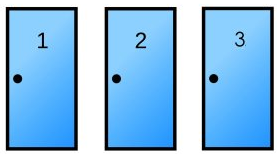You’ll get the prize behind the door you pick, but you don’t know which prize is behind which door. Obviously you want the car!

So you pick, for example, door A. Before opening your door, the host, who knows what’s behind the doors, opens another door, say No. C, which has a goat.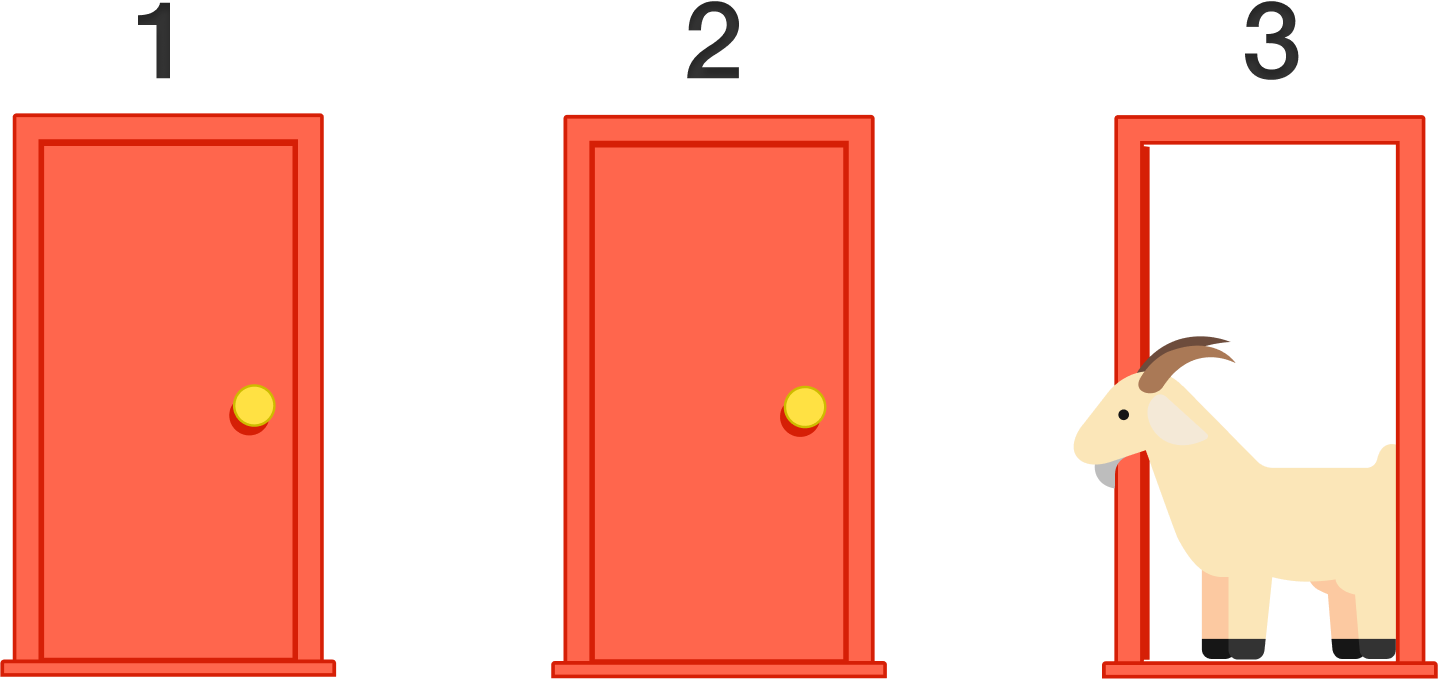Note that Monty needs to open a door and that he will never open the door that has the car.

He then asks you if you’d like to change your guess. Should you?

A lot of people think that it doesn’t matter if I change my guess or not. There are 2 doors, so the odds of winning the car with each is 50%. Unfortunately, that’s 100% wrong. Using Bayes theorem, we can prove why it is in your best interest to change doors.

Assuming that we picked door A and Monty opened door C, we need to calculate 2 posteriors.

1. $$\mathbb{P}(\text{door}=A|\text{opens}=C)$$, the probability of $$A$$ is correct if Monty opened C,
2. $$\mathbb{P}(\text{door}=B|\text{opens}=C)$$, the probability of $$B$$ is correct if Monty opened $$C$$.

### Prior: P(A)

The probability of any door being correct before we pick a door is $$\frac{1}{3}$$.

• $$\mathbb{P}(\text{door}=A) = \frac{1}{3}$$
• Prior probability that door A contains the car
• $$\mathbb{P}(\text{door}=B) = \frac{1}{3}$$
• Prior probability that door B contains the car

### Likelihood: P(B|A)

If the car is actually behind door A, then Monty can open door B or C. So the probability of opening either is $$\frac{1}{2}$$.

If the car is actually behind door B then Monty can only open door C as he can’t open A, the door we picked and also can’t open door B because it has the car behind it.

• $$\mathbb{P}(\text{opens}=C \mid \text{door}=A) = \frac{1}{2}$$
• Likelihood Monty opened door C if door A is correct
• $$\mathbb{P}(\text{opens}=C \mid \text{door}=B) = 1$$
• Likelihood Monty opened door C if door B is correct

### P(B)

We can calculate $$\mathbb{P}(C)$$ as:

\begin{align*} \mathbb{P}(\text{door} = C) & = \mathbb{P}(C \mid A) \mathbb{P}(A) + \mathbb{P}(C\mid B)\mathbb{P}(B) \\ & = \frac{1}{2}\times \frac{1}{3}+1\times \frac{1}{3} = \frac{1}{2} \end{align*}

### Posterior: P(A|B)

Now we do the remaining math:

\begin{align*} \mathbb{P}(\text{door}=A \mid \text{opens}=C) & = \frac{\mathbb{P}(\text{open}=C \mid \text{door} = A)\mathbb{P}(\text{door=A})}{\mathbb{P}(\text{open=C})} \\ &= \frac{\frac{1}{2}\times \frac{1}{3}}{\frac{1}{2}} = \frac{1}{3} \end{align*}

and

\begin{align*}\mathbb{P}(\text{door}=B \mid \text{opens}=C) &= \frac{\mathbb{P}(\text{open}=C \mid \text{door} = B)\mathbb{P}(\text{door=B})}{\mathbb{P}(\text{open=C})} \\ &= \frac{1 \times \frac{1}{3}}{\frac{1}{2}} = \frac{2}{3} \end{align*}

This leaves us with a with a higher probability of winning if we change doors after Monty opens a door.##### Emmanuelle R. Nunes
###### Senior Data Scientist

My interests include statistics, machine learning, health and social statistics.